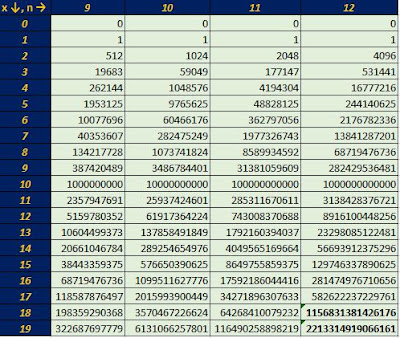## Saturday, July 11, 2015

### Powers of Integers: Last Digit

Powers of Integers: Last Digit

Let x^n where x is a non-negative integer (x = 0, 1, 2, 3, …) and n is a positive integer (n = 1, 2, 3, …).

Starting with n = 1, note the last digit of each integer.  A table of where 0 ≤ x ≤ 19 and 0 ≤ n ≤ 12 is shown below:x^n:  x = 0 to 19, n = 0 to 12
Note:  The numbers in bold I typed in because the number of digits Excel 2013 will carry in its integers will only go so far.

Now, let’s look at just the last integer.  On Excel, the last digit can be extracted by the following formula:

=number-ROUNDOWN(number/10,0)*10Last digit of x^n

Starting to see a pattern?  I know this is not a rigorous proof, but four patterns definitely present themselves:

For 1 ≡ n mod 4  (n = 1, 5, 9…), the last digits of x^n follow this pattern:
0, 1, 2, 3, 4, 5, 6, 7, 8, 9

For 2 ≡ n mod 4  (n = 2, 6, 10, …), the last digits of x^n follow this pattern:
0, 1, 4, 9, 6, 5, 6, 9, 4, 1

For 3 ≡ n mod 4 (n = 3, 7, 11, …), the last digits of x^n follow this pattern:
0, 1, 8, 7, 4, 5, 3, 6, 2, 9

And for 0 ≡ n mod 4  (n = 4, 8, 12, ….), the last digits of x^n follow this pattern:
0, 1, 6, 1, 6, 5, 6, 1, 6, 1

Eddie

This blog is property of Edward Shore.   2015.